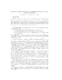## Stable Factorizations of Symmetric Tridiagonal and Triadic MatricesWe call a matrix triadic if it has no more than two nonzero off-diagonal elements in any column. A symmetric tridiagonal matrix is a special case. In this paper we consider $LXL^T$ factorizations of symmetric triadic matrices, where $L$ is unit lower triangular and $X$ is diagonal, block diagonal with $1\!\times\!1$ and $2\!\times\!2$ blocks, or the identity with $L$ lower triangular. We prove that with diagonal pivoting, the $LXL^T$ factorization of a symmetric triadic matrix is sparse, study some pivoting algorithms, discuss their growth factor and performance, analyze their stability, and develop perturbation bounds. These factorizations are useful in computing inertia, in solving linear systems of equations, and in determining modified Newton search directions.